×

Data

# CASE Statements vs. IF Statements in Tableau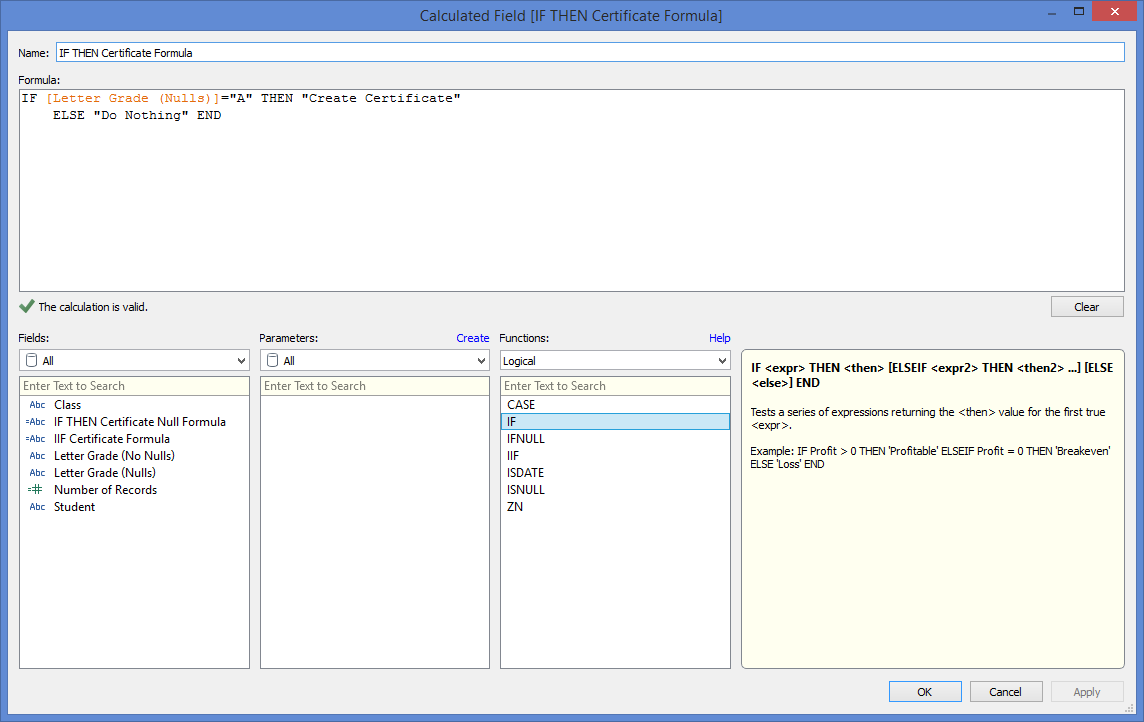//

What are the differences between CASE and IF statements when making calculated fields in Tableau? How do different types of IF statements vary? When is it better to use one of these statements over the others? That is what I’ll address in this article.

## The Different Types of IF Statements

Before discussing CASE statements, it is important to point out the different types of IF statements in Tableau. There is the classic IF THEN statement with the ability to add multiple ELSEIF components. There is also the IIF statement, which handles unknown results differently and uses a different syntax. To illustrate these and other differences throughout this article, I’ll use a simple data set of student grades.

Let’s say that for every “A” grade earned, a certificate needs to be issued. And, I want a calculated field to identify these instances. I could use a simple IF THEN statement (Figure 1) or an IIF statement (Figure 2).Figure 1: This formula separates the boolean results into two categories: TRUE and everything else (FALSE and Unknown).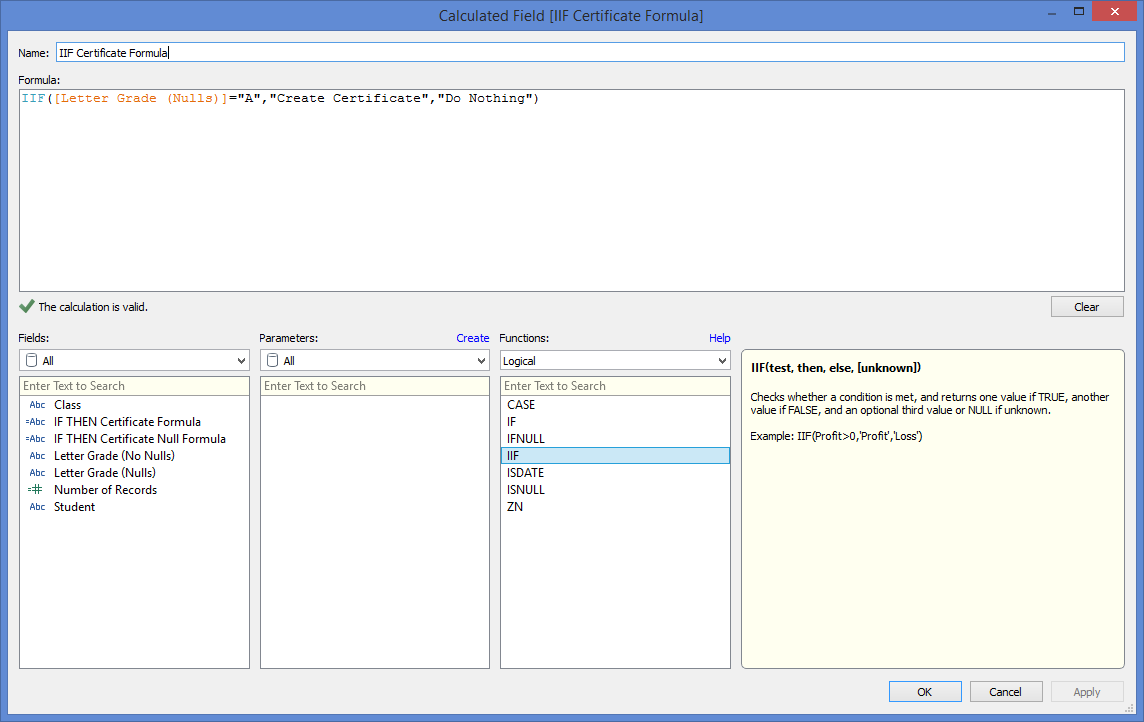Figure 2; This formula separates the boolean results into three categories: TRUE, FALSE, and UNKNOWN. When an UNKNOWN value is not specified, the formula will return null  for that row.

Depending on the data, syntax might be the only difference between these two. If so, the IIF statement is simpler and less prone to making errors. However, it might not be as readable if the user is not familiar with it. The primary difference between these two statements can be seen when the data contains values that yield an “unknown” result from the boolean comparison. This typically happens when there are null values in the data set.

In this case, the IF THEN unknown results are treated and given the same value as the false results whereas the IIF statement returns “null” or another specified value for unknown results. In our example, let’s say that the math teacher forgot to enter some grades. The IF THEN formula would assign those rows with missing grades a “Do Nothing” value, but the IIF formula would leave them null. Figure 3 shows this in a crosstab with the differences highlighted.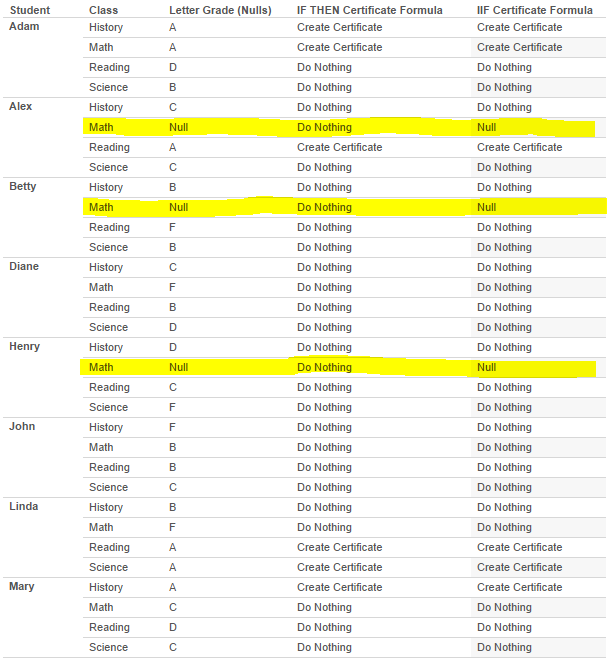Figure 3: When IF THEN statements do not account for unknown results, they can misrepresent the data as shown in this example.

As you can see, the IIF calculation reports the data more accurately and points out when there are nulls. In this way, the IIF statement is superior to the IF THEN statement. There is an easy workaround to this problem with the IF THEN statement using an ISNULL function, but it’s messier (see Figure 4).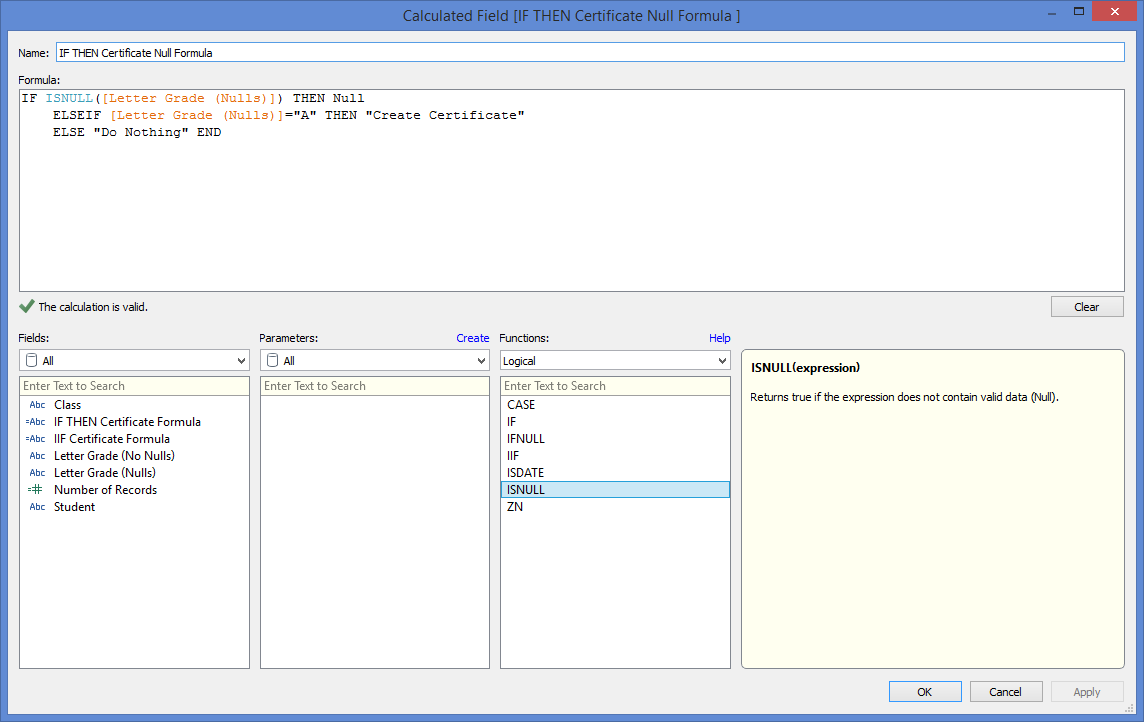Figure 4: IIF THEN statements can properly account for UNKNOWN results by using functions like the ISNULL shown in this formula.

IF THEN statements, however, are far better than IIF statements when function nesting is necessary. For example, let’s say that I want to translate all the A-F grades into a numerical grade in order to compute their GPAs. The IF THEN formula is fairly straightforward and easy to understand (Figure 5). The IIF formula in Figure 6 is much more difficult to write and understand.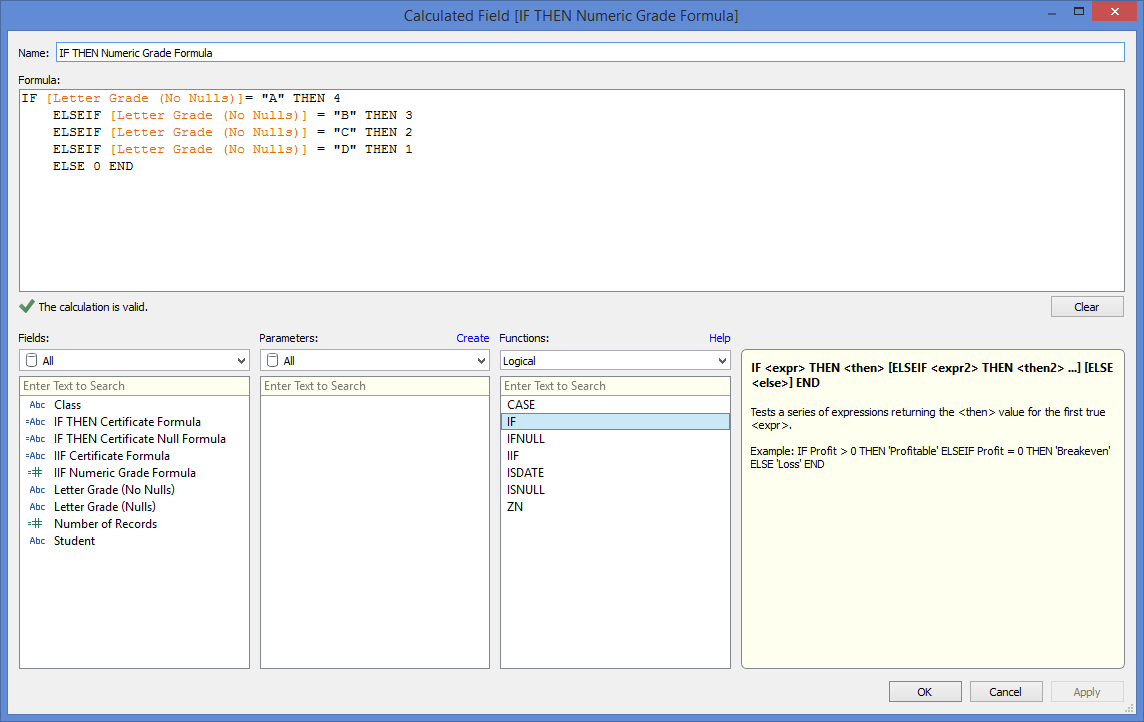Figure 5: Using IF THEN ELSEIF… is usually the best method for nesting these kinds of functions.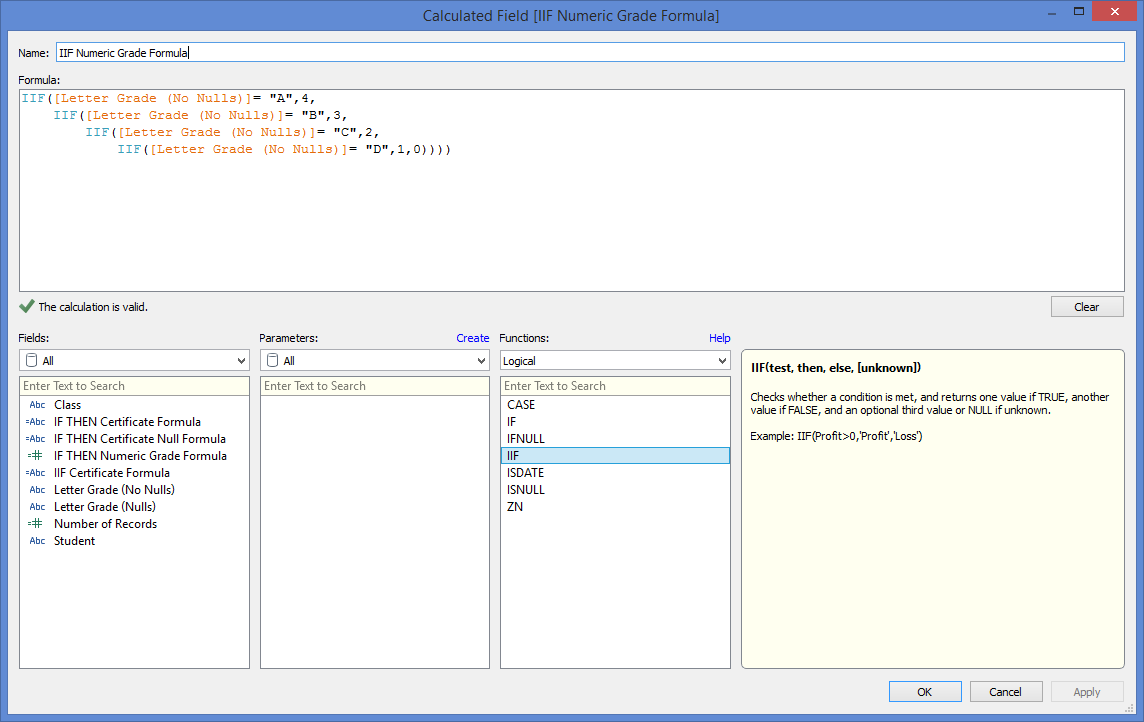Figure 6: While this formula is valid, it is a really bad method for performing this calculation!

So, when it comes to IF statements in Tableau, use the IIF statement when the calculation is simple or if you’re not sure about the data quality of the field you’re testing. Many times though, you’re better off using the usual IF THEN statement; just make sure that your formula is correctly handling unknown results.

## Comparing to CASE Statements

For the purpose of comparing CASE statements to the IF statements, I will use the IF THEN type of IF statement. For many calculations, both CASE and IF statements can be used to get the same result. For example, to translate the letter grades into numerical grades, as shown above, the CASE formula in Figure 7 will also work!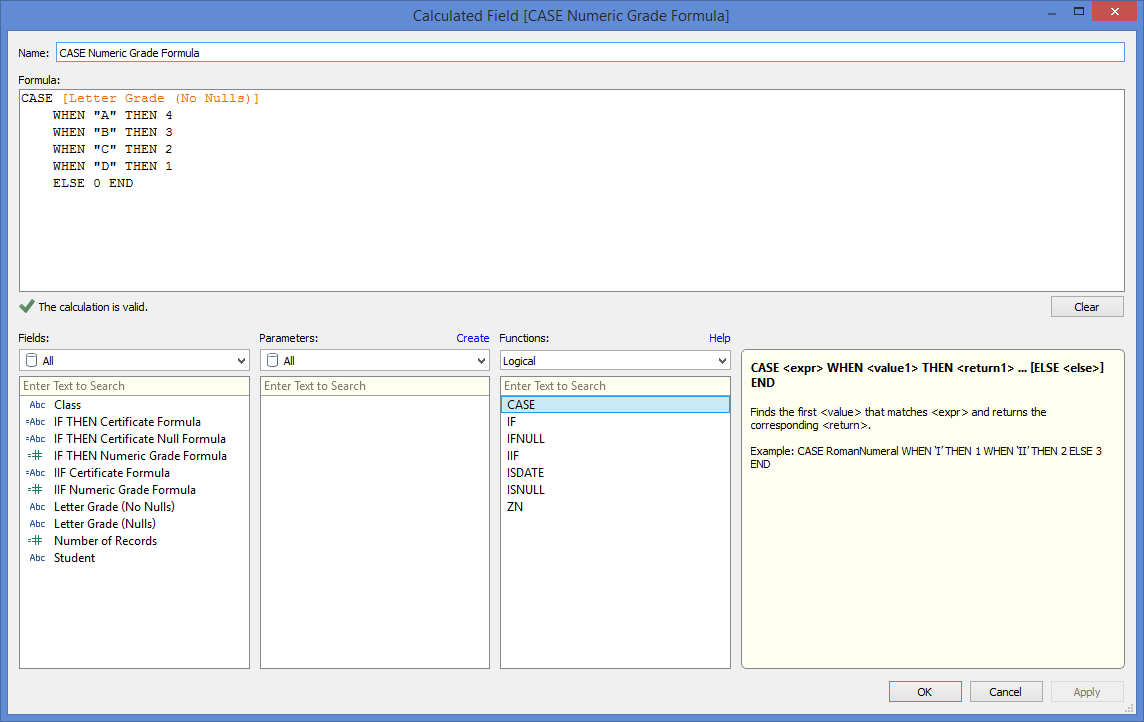Figure 7: The ELSE clause is optional. Without it, any unmatched results will be considered null.

In this example, we see one of the advantages of sometimes using a CASE statement: simplicity. In this example, not only is the CASE statement easier to understand, but it is also easier to avoid making mistakes like referencing the wrong field. The other advantage of CASE statements over IF statements is that CASE statements are faster. This is because they function as a sort of switch statement.

However, CASE statements in Tableau are significantly limited because they cannot perform boolean algebra! This can be a point of confusion for someone familiar with SQL CASE statements that are not thus inhibited. The restriction on Tableau’s CASE statements makes it extremely difficult or impossible to use them to perform many logical calculations.

This is where the ability of Tableau’s IF statements to perform boolean algebra makes them far more useful than CASE statements. Let’s say I want a calculated field that classifies the students into three groups according to their GPA: Advanced, Average and Below Average. The IF statement in Figure 8 easily accomplishes this.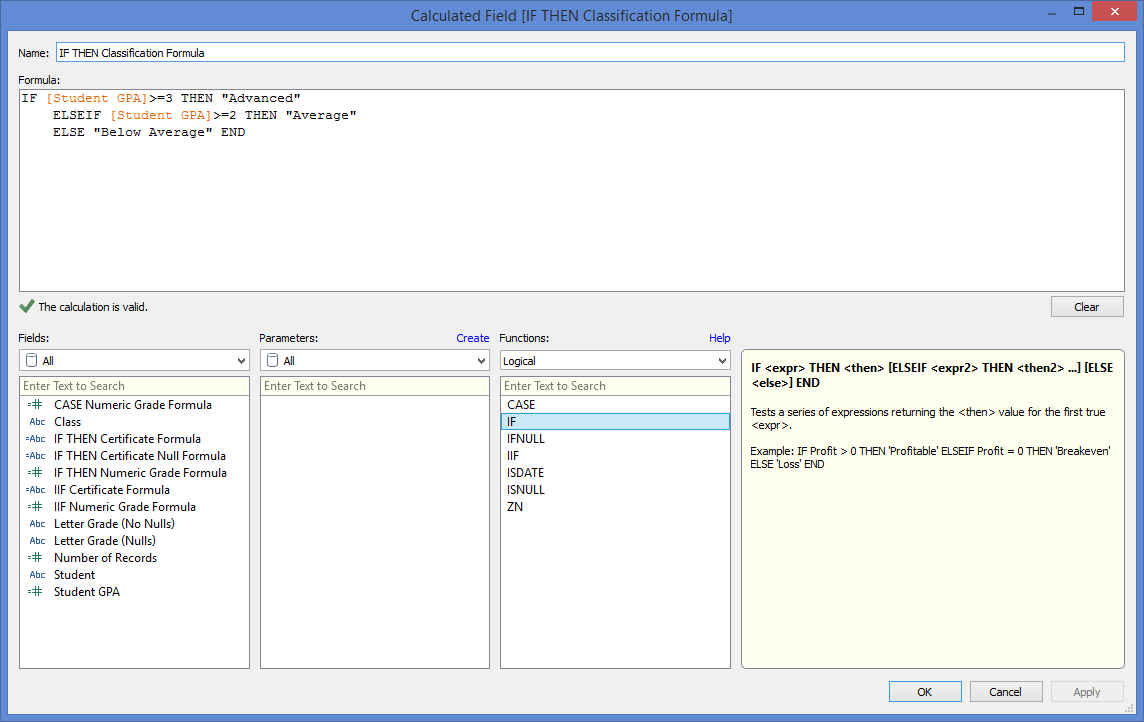Figure 8: Remember that IF THEN statements require boolean expressions – expressions that can be evaluated as either TRUE of FALSE.

But CASE statements can’t perform this calculation in Tableau because they can only compare the expression to the exact string values you list. Another advantage of IF statements over CASE statements is that IF statements are simpler when wanting to evaluate multiple expressions in a single calculated field.

Let’s say a teacher is trying to start a new STEM club for girls and wants to create a calculated field that separates students who are both female and academically eligible from the rest of the students. With IF statements, the formula is very simple (see Figure 9). But, you see in Figure 10 that doing this with CASE statements gets messy because it requires nested statements.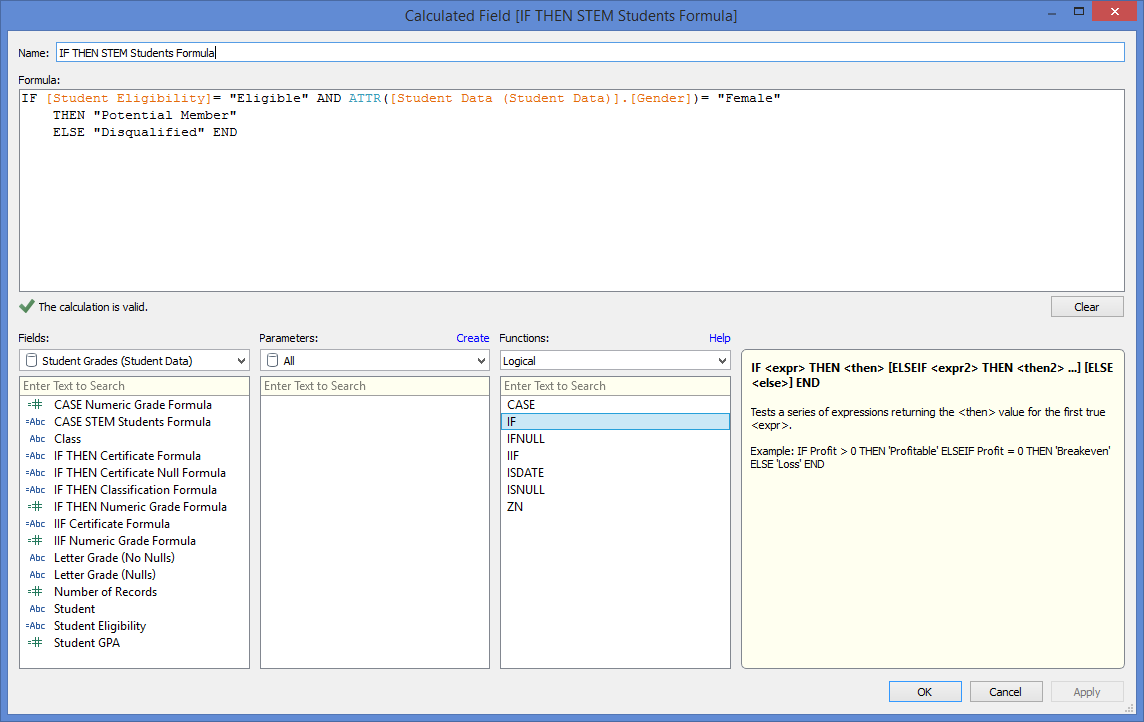Figure 9: Where possible, try to use logical operators like AND. They can make simplify formula structures and boost performance.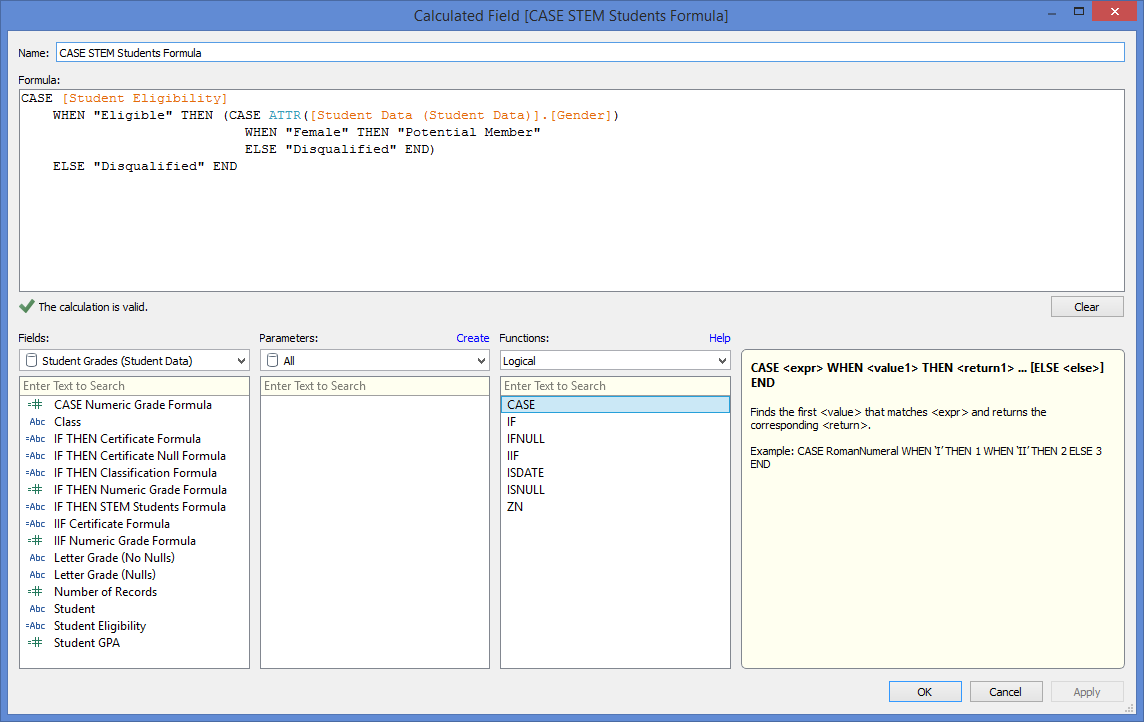Figure 10: Not only is nesting with CASE statements hard to read, it is also easy to make mistakes. Try to avoid CASE nesting like in this example wherever possible.

## The Final Verdict

In summary, if your calculation is a choice between a simple CASE statement and an IF statement, go with the CASE statement. It’s clearer and will perform better. But, if you’re using a CASE statement and keep getting an error, or you find yourself writing some complicated formula, then you’re probably better off using an IF statement.

### The Latest

• ##### Tableau Cloud System (SCIM): Unifying the Dimensions

It’s in Our DNA: The InterWorks Difference I regularly hear from my friends and family how awesome they think my job is. I wholeheartedly agree! I started at InterWorks in ...
How I Learned Tableau Prep Is Awesome I don’t blog often, but when I do, I blog about something awesome. This is the case with Tableau Prep. Tableau Prep has been out for a ...

See more from this author →

InterWorks uses cookies to allow us to better understand how the site is used. By continuing to use this site, you consent to this policy. Review Policy OK

×

Interworks GmbH
Ratinger Straße 9
40213 Düsseldorf
Germany
Geschäftsführer: Mel Stephenson

Kontaktaufnahme: markus@interworks.eu
Telefon: +49 (0)211 5408 5301

Amtsgericht Düsseldorf HRB 79752
UstldNr: DE 313 353 072